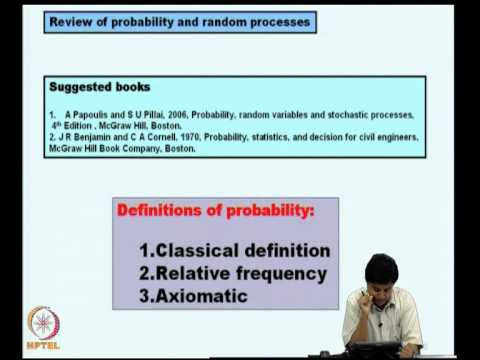### Stochastic Structural DynamicsThe objective of this course is to develop methods for analysis of structures subjected to dynamic loads which are random in nature.
Structures under the action of wind or earthquake loads are typical of such problems. The course introduces the application of probability, random variables and random processes to model uncertainties in dynamic loads.
The response analysis considers question of propagation of uncertainties in the inputs to the response variables of interest and also considers questions on reliability of vibrating systems under dynamic loads.
The course mainly deals with linear time invariant systems. A brief introduction to random vibration analysis of nonlinear systems is also provided. Solution strategies include analytical techniques and Monte Carlo simulation procedures.

 Sl. No. Topic/s Number of Hours 1. Motivations. Probability space. Conditional probability. Random variables. Probability distribution and density functions; expectations. Commonly occurring random variables. Sequence of random variables and limit theorems. 8 2. Random processes and classifications. Stationary and ergodicity. Power spectral density function and auto - covariance functions. Gaussian, Poisson and Poisson pulse processes. Mean square derivatives and integrals. Examples on models for earthquake and wind loads. 8 3. Review of input output relations in time and frequency domains for linear time invariant systems. Uncertainty propagation under stationary and nonstationary excitations. SDOF and MDOF systems. 8 4. Structural failure under random vibrations. Level crossing problems. First passage time. Models for peaks and envelopes. Extreme value distributions. Narrow band processes and clumping effects. 8 5. Applications to response spectrum based methods in earthquake engineering and gust factor approach in wind engineering. 2 6. Fatigue failure. Estimation of expected life time under random loading. 2 7. Introduction to Monte Carlo simulation methods. Simulation of random variables and random processes. Problem of variance reduction. Application to models for uncertainty propagation and reliability analysis. 4 Total Hours 40